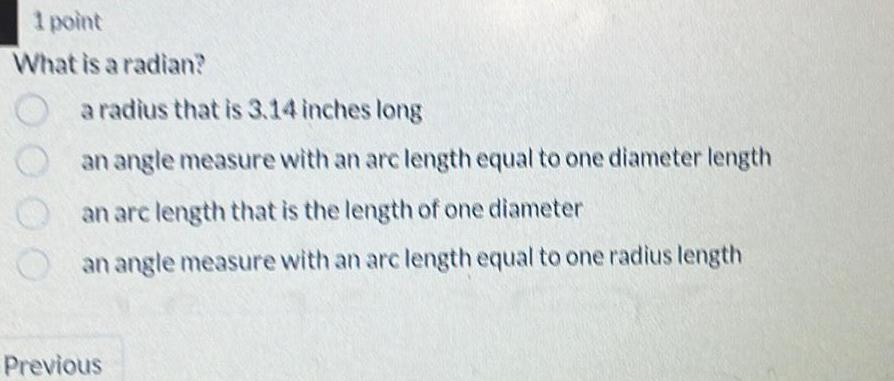Question:

# 1 point What is a radian a radius that is 3 14 inches long

Last updated: 9/20/20231 point What is a radian a radius that is 3 14 inches long an angle measure with an arc length equal to one diameter length an arc length that is the length of one diameter an angle measure with an arc length equal to one radius length Previous